Next: TOTAL ESCAPE PROBABILITY FOR Up: thesis Previous: ALGORITHMS   Contents

# PATHWAY SUMS FOR CHAIN GRAPHS, #MATH944#

To obtain the total probability of leaving the chain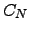via node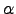if started from node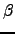, i.e.  , we must calculate the pathway sum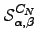. We start with the case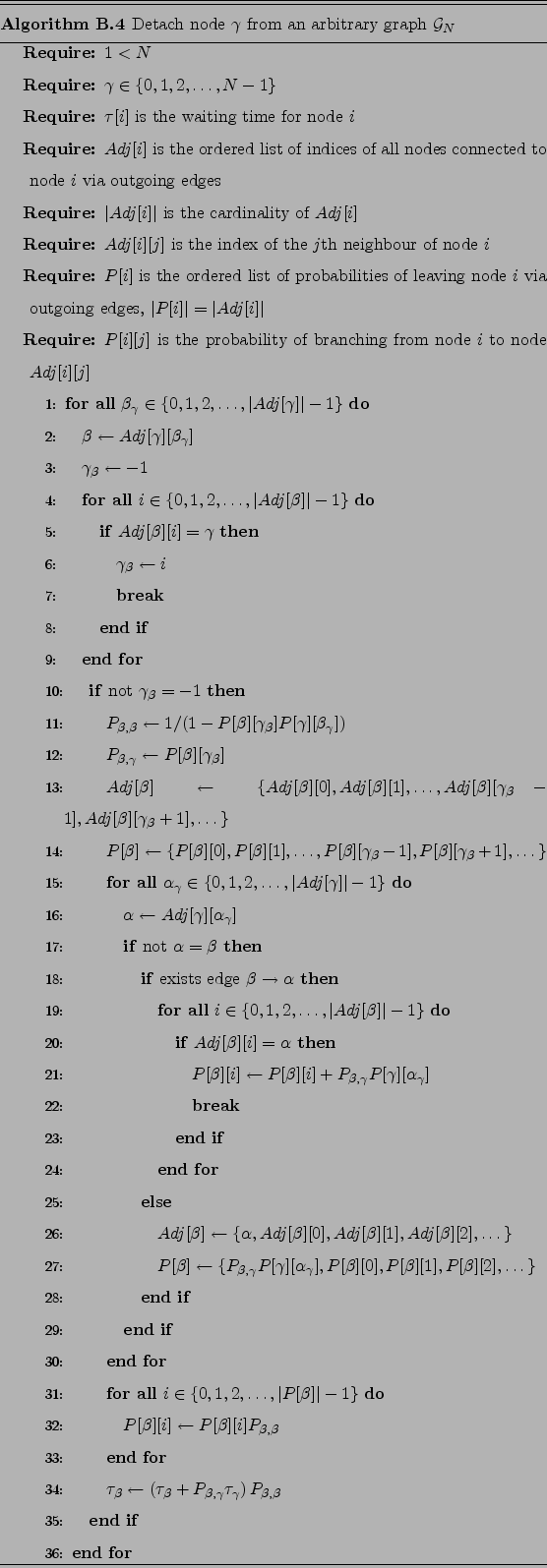and obtain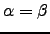. Consider any path that has reached node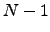. The probability factor due to all possibleto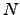recrossings is simply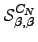. We need to include this factor every time we reach nodeduring recrossings of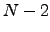to. The corresponding sum becomes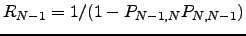(C.1)

Similarly, we can continue summing contributions in this way until we have recrossings ofto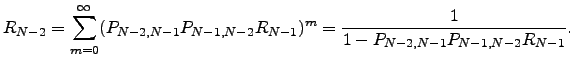, for which the result of the nested summations is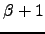. Hence,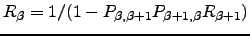is the total transition probability for pathways that return to nodeand are confined to nodes with index greater thanwithout escape from.

We can similarly calculate the total probability for pathways returning toand confined to nodes with indices smaller than. The total probability factor for recrossings between nodes 1 and 2 is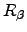. Hence, the required probability for recrossings between nodes 2 and 3 including arbitrary recrossings between 1 and 2 is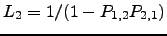. Continuing up to recrossings between nodes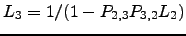andwe obtain the total return probability for pathways restricted to this side ofas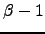. The general recursive definitions of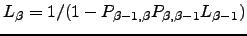and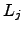are: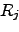(C.2)

We can now calculateas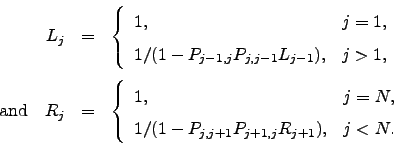(C.3)

where we have used Equation C.2 and the multinomial theorem .

We can now deriveas follows. If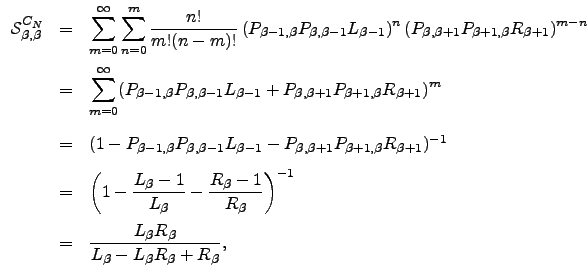we can write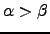(C.4)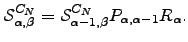gives the total transition probability fromto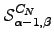, so the corresponding probability for nodeistimes the branching probability fromto, i.e.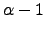, times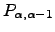, which accounts for the weight accumulated from all possible paths that leave and return to nodeand are restricted to nodes with indexes greater than. We can now replaceby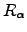and so on, untilis expressed in terms of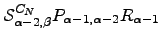. Similarly, ifwe have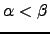(C.5)

and hence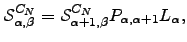(C.6)Next: TOTAL ESCAPE PROBABILITY FOR Up: thesis Previous: ALGORITHMS   Contents
Semen A Trygubenko 2006-04-10